# The Malstrm Audio Inputs

### The Malström Audio Inputs

This brings us to the last example of the Malström section. It's time to try something fancier: using the Malström filter audio inputs. You can hook other devices to the shaper and to the other filter types like Comb+/- and AM.

It also means you'll need to create one new instrument for each input. To do this in the final Malström exercise, we'll use two SubTractors playing plain old init patches.

#### To hook external synths through the Malström

1.

Cable each of the Malström's oscillator outputs to a separate Mixer track (Figure 5.126). Just click the output and drag to the input you want.

##### Figure 5.126. Instruments plugged into the Malström audio inputs will need the Filter A and B outputs to pass sound on to the Mixer.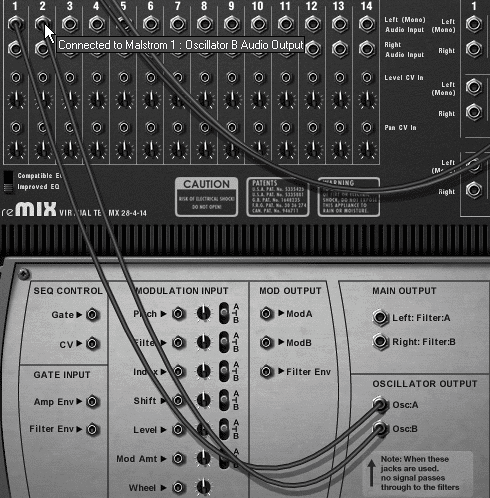These will be used to play a Malström melody.

2.

Write a basic two-bar sustained melody loop for the Malström (Figure 5.127).

##### Figure 5.127. Example of a basic two-bar sustained melody loop.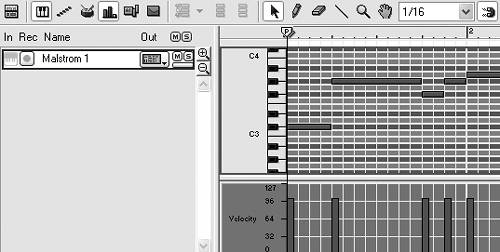3.

Hold down the Shift key to disable auto-routing, and create two SubTractor synths. Without auto-routing, you'll be cabling these manually.

4.

Go to the back panel and cable one SubTractor audio out to the Malström's Shaper/Filter:A audio input, and the other to the Malström's Filter:B input (Figure 5.128).

##### Figure 5.128. Hook two SubTractor synths to the Maelstrom's audio inputs.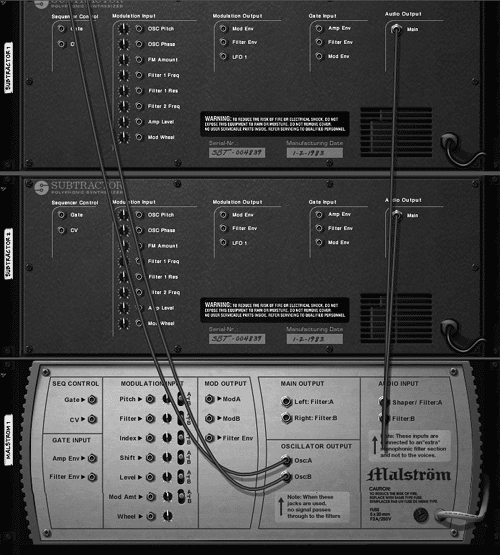5.

Minimize the SubTractors by clicking the fold/unfold arrows.

6.

Now cable the Malström's main left and right outputs to separate Mixer channels (Figure 5.129). These will be outputting the filtered SubTractor tracks.

##### Figure 5.129. Hook up the Malström Filter A and B outputs to pass the SubTractor signals out the Malström to the Mixer.7.

Now preserve your work by saving your song somewhere easy to find. (Saving a patch won't keep your cabling!)

Now let's set up the Malström controls to use on the SubTractor signals. We're going to use the Malström's Mod B to move Filter A and its filter envelope to move Filter B.

#### To run external synths through the Malström filters

1.

In your Sequencer, write a simple two-bar, sixteenth-note pulse line for SubTractor 1 (Figure 5.130).

##### Figure 5.130. A simple, pulse bass line is sufficient for testing the Malström audio input signal.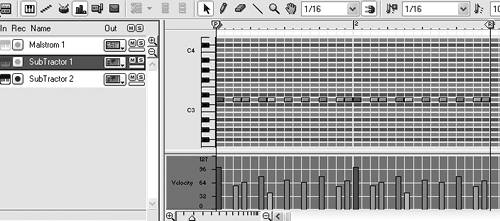2.

Activate the Arrange view. Then copy and drag the SubTractor 1 track to the SubTractor 2 track (Figure 5.131).

##### Figure 5.131. Drag-copy the bass track to the second SubTractor in the Arrange window.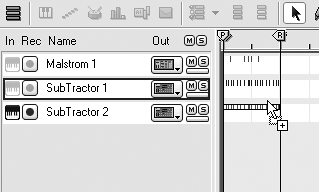This is one fast way to get two SubTractor synths to play the same notes.

3.

4.

Set Filter A type to Comb+, set the frequency to 52, and the resonance to 66 (Figure 5.132).

##### Figure 5.132. This is a good setting for a moving filter.This is a midrange setting good for a moving filter.

5.

To control Filter A with Mod B, set Mod B's destination to A and turn the Mod B Filter knob to 42 (Figure 5.133).

##### Figure 5.133. Set Mod B to move Filter A.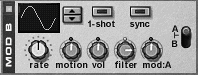This causes Filter A's frequency to rise according to the Mod B shape.

6.

Set the Mod B curve to 4 (positive square), turn Sync on, and set the Rate knob to 1/4 (Figure 5.134).

##### Figure 5.134. Set the Mod B curve and rate parameters.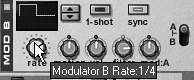Since Filter A is set at fairly high resonance and is creating some harmonic tone, this will have the effect of imposing a ping-pong pitch shift on it.

7.

Enable Filter B and set the type to LP 12.

8.

To get Filter B to respond to the filter envelope, click Filter B's Env button.

9.

Set the Filter B frequency to 52 and the resonance to 71 (Figure 5.135).

##### Figure 5.135. Set Filter B with a medium frequency and boost its resonance.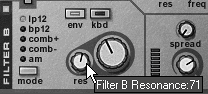10.

Set the filter envelope Amt knob to 30 (Figure 5.136).

##### Figure 5.136. Filter envelope settings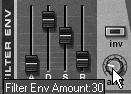If you want more filter motion than this, try boosting the filter envelope Amt knob more and bringing the Filter B Freq knob down.

11.

Save the patch in a likely, easy-to-reach spot.

For the last task, we'll set up the Malström's voice. We're already using the Malström's filters and Mod B for the SubTractor voices, which leaves us with just Mod A. That's plenty to create a long phasing effect that will sound good next to the SubTractor's pulsing lines.

#### To use Mod A on the Malström oscillators

1.

Set the Malström's Osc A to Sawtooth*4. Turn the Motion knob to 40 and move the Index slider to a harmonically rich 57 (Figure 5.137).

##### Figure 5.137. Change the Osc A Index setting.2.

Choose Square for the Malström's Osc B, and turn Motion to 40 (Figure 5.138).

##### Figure 5.138. Set Osc B's motion fairly slow.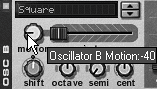This sets up slow voices that complement each other and will respond well to Mod A Index and Shift modulations.

3.

Enable Mod A.

4.

Set the Mod A destination to A and B (the middle).

5.

Turn the Mod A Index knob to 36.

6.

Turn on the Mod A Sync and set the Rate to 8/4 (Figure 5.139). This sets up Mod A to give us a fairly subtle tonal change.

##### Figure 5.139. Set Mod A to a fairly slow rate for a more subtle effect.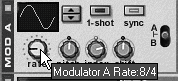7.

Save your patch if you think you might want to use it again!Tips

• For some nice variations on the sound created in this exercise, try changing the Filter A frequency, the Mod B Filter knob, and the filter envelope's Amt knob.

• Try loading some patches from the Factory Sound Bank into your SubTractors, or tuning the oscillators for some fatter tones.Reason 3 For Windows and Mac
ISBN: 321269179
EAN: N/A
Year: 2003
Pages: 180